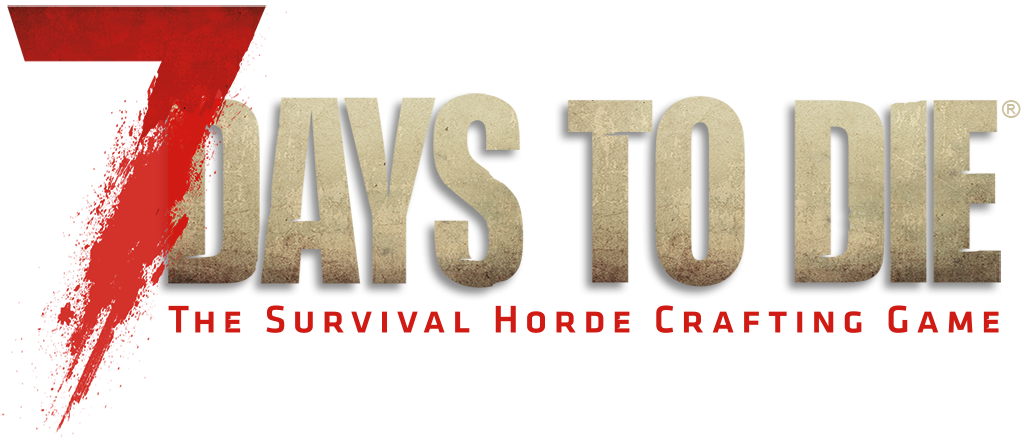Jump to content## Recommended Posts

I searched, but couldn't find definitive answers.

I'd like to know how ranged values work in the XML / Xpath Modding

Example 1

`<passive_effect name="EntityDamage" operation="perc_add" value="1,5" tier="2,6"/>`

I know basically what this does, but it's the ranged values I'm unsure about.

What will the effect be?

1. Will this Add a random percent (Between 1 and 5) to "EntityDamage" for all items that are Tier 2 - 6? (So a Tier 2 could get 5% while a Tier 6 might get 1%?)
2. Will this split the values evenly always giving a Tier 2 Item 1%, A Tier 3 Item 2% etc... Up to a Tier 6 Item at 5%? (So Tier 2 is always lower than Tier 3?)
3. Will this just be random like # 1 but but just more likely to give the lower Tier a lower %?

So I guess based on the answer above I'll know if the example below is the same thing?

Example 2

`<passive_effect name="EntityDamage" operation="perc_add" value="1,2,3,4,5" tier="2,3,4,5,6"/>`

I know this should mean strictly that:

Tier 2 = 1%,

Tier 3 = 2%,

Tier 4 = 3%,

Tier 5 = 4%,

Tier 6 = 5%

What happens if the ranges dont match?

Example 3

```<passive_effect name="EntityDamage" operation="perc_add" value="1,2,3" tier="2,3,4,5,6"/>
or

##### Share on other sites

the first one you mentioned is a random set from 1 to 5 for tiers 2-6. So it will throw a dice each time new item appears to give you the value.

the second one is for strict setting value/tier: 1/2, 2/3, 3/4, 4/5, 5/6

for you question:

I assume that strict ranges use arrays under the hood, so you will end up having : 1/2, 2/3, 3/4, null/5, null/6 - for the first line (Possible NPE if it is not covered by C# variation of Optional.ofNullable(n).orElse(0))

and a regular random from 1 to 5 for the tiers 2,3,6 for the second example

##### Share on other sites

I would say the answer to your first question is option 2, but instead of 1% it would be 100%. 0.01=1%, 0.1=10%, 1=100%

Lets look at an actual example, the iron reinforced club:

```<passive_effect name="EntityDamage" operation="base_set" value="17.4"/> <!-- meleeClubIron -->
...
<passive_effect name="EntityDamage" operation="perc_add" value="-.15,.15"/> <!-- random EntityDmg -->
<passive_effect name="EntityDamage" operation="perc_add" value=".1,.5" tier="2,6"/> <!-- tier bonus -->
```

So the first line sets a base value for quality 1 at 17.4.

The second line adds a random percentage between -15% and +15%. 15% is 2.61

The third line adds 10% for quality 2, 20% for quality 3, ... 40% for quality 5 items and 50% for quality 6. (Note the xml says tier for quality)

So a quality 1 iron reinforced club should be between 14 and 20, a quality 5 between 21 (=17.4*125%) and 26 (=17.4*155%), a quality 6 between 23 and 28.

So I did a test, got myself a few workbenches and produced 18 quality 1 iron reinforced clubs. I got a range of values between 14 and 19. The value 20 is very difficult to get, a 20 should be 100 times as seldom as a 15 for example (as the random value has to land between 20 and 20.1, while it will be a 15 between 15 and 15.99).

Then produced 14 quality 5 iron reinforced clubs and got values between 21 and 26.

Ok, the theory fits reality very well I would say.

#### Archived

This topic is now archived and is closed to further replies.

×

• #### Support

• Discord
• Guilded
×
• Create New...